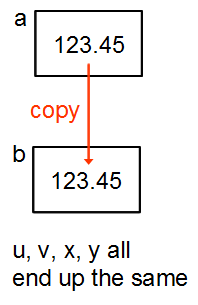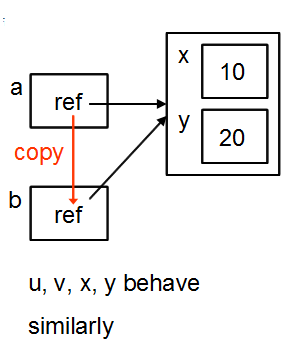## Variables holding valuesThis applies to primitive types: Number and Boolean.

### Copying

``````
var a = 123.45;
var b = a;
``````

There are then 2 separate variables holding the same value - end of story, nothing remarkable.

### Passing as parameters

``````
var u = 123.45;
var v = f (u);

function f (x) // x is a copy of u
{
var y = x;
return y;
} // x & y then thrown away
``````

### Comparing

``````
if (a == b) ... // compare values
``````

### Variables holding referencesThis applies to objects and arrays. The variable holds something that enables the system to find the object. We call that something a "reference". (Java programmers will be familiar with this. C/C++ programmers would call it a pointer except that you have no access to it to change where it points - many of us think that is a very good thing.)

### Copying

``````
var a = { x:10; y:20 };
var b = a;
``````

An object is created somewhere in memory, holding the values of the 2 properties x and y. Variable a contains a reference to that memory location, in a form which the system can use but we cannot. The assignment to b copies the reference so that then both a and b refer to the same object.

### Passing as parameters

``````
var u = { x:10; y:20 };
var v = f (u);

function f (x)
{ // x is copy of reference
var y = x;
return y;
} // x & y then thrown away
``````

At the end of all that v is again a reference to the same object as u, in this particular case.

### Comparing

``````
if (a == b) ... // compare references
``````

Are the references the same? Are both a and b referring to the same location in memory?

NB: There is no `equals()` function, unlike other languages. Instead we have to compare every property of 2 distinct objects to see whether they can be considered to be equal.

## Summary table

TypeCopyingParametersComparison
NumberValueValueValue
BooleanValueValueValue
StringImmutable*Immutable*Value
ObjectReferenceReferenceReference
ArrayReferenceReferenceReference

* The fact that Strings are immutable means it is impossible to tell how they are copied or passed into functions, so it doesn't matter.

NB: Compare strings with s == t, unlike Java. They are compared by value.